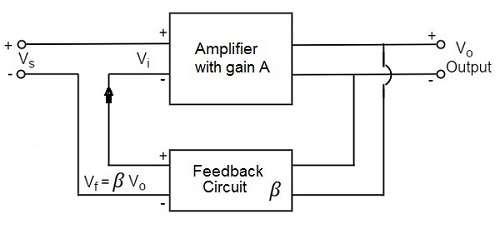# Amplifiers Feedback

Advertisements

An amplifier circuit simply increases the signal strength. But while amplifying, it just increases the strength of its input signal whether it contains information or some noise along with information. This noise or some disturbance is introduced in the amplifiers because of their strong tendency to introduce hum due to sudden temperature changes or stray electric and magnetic fields. Therefore, every high gain amplifier tends to give noise along with signal in its output, which is very undesirable.

The noise level in the amplifier circuits can be considerably reduced by using negative feedback done by injecting a fraction of output in phase opposition to the input signal.

## Principle of Feedback Amplifier

A feedback amplifier generally consists of two parts. They are the amplifier and the feedback circuit. The feedback circuit usually consists of resistors. The concept of feedback amplifier can be understood from the following figure.From the above figure, the gain of the amplifier is represented as A. the gain of the amplifier is the ratio of output voltage Vo to the input voltage Vi. the feedback network extracts a voltage Vf = β Vo from the output Vo of the amplifier.

This voltage is added for positive feedback and subtracted for negative feedback, from the signal voltage Vs. Now,

$$V_i = V_s + V_f = V_s + \beta V_o$$

$$V_i = V_s - V_f = V_s - \beta V_o$$

The quantity β = Vf/Vo is called as feedback ratio or feedback fraction.

Let us consider the case of negative feedback. The output Vo must be equal to the input voltage (Vs - βVo) multiplied by the gain A of the amplifier.

Hence,

$$(V_s - \beta V_o)A = V_o$$

Or

$$A V_s - A \beta V_o = V_o$$

Or

$$A V_s = V_o (1 + A \beta)$$

Therefore,

$$\frac{V_o}{V_s} = \frac{A}{1 + A \beta}$$

Let Af be the overall gain (gain with the feedback) of the amplifier. This is defined as the ratio of output voltage Vo to the applied signal voltage Vs, i.e.,

$$A_f = \frac{Output \: voltage}{Input \: signal \: voltage} = \frac{V_o}{V_s}$$

So, from the above two equations, we can understand that,

The equation of gain of the feedback amplifier, with negative feedback is given by

$$A_f = \frac{A}{1 + A \beta}$$

The equation of gain of the feedback amplifier, with positive feedback is given by

$$A_f = \frac{A}{1 - A \beta}$$

These are the standard equations to calculate the gain of feedback amplifiers.

## Types of Feedbacks

The process of injecting a fraction of output energy of some device back to the input is known as Feedback. It has been found that feedback is very useful in reducing noise and making the amplifier operation stable.

Depending upon whether the feedback signal aids or opposes the input signal, there are two types of feedbacks used.

### Positive Feedback

The feedback in which the feedback energy i.e., either voltage or current is in phase with the input signal and thus aids it is called as Positive feedback.

Both the input signal and feedback signal introduces a phase shift of 180o thus making a 360o resultant phase shift around the loop, to be finally in phase with the input signal.

Though the positive feedback increases the gain of the amplifier, it has the disadvantages such as

• Increasing distortion
• Instability

It is because of these disadvantages the positive feedback is not recommended for the amplifiers. If the positive feedback is sufficiently large, it leads to oscillations, by which oscillator circuits are formed. This concept will be discussed in OSCILLATORS tutorial.

### Negative Feedback

The feedback in which the feedback energy i.e., either voltage or current is out of phase with the input and thus opposes it, is called as negative feedback.

In negative feedback, the amplifier introduces a phase shift of 180o into the circuit while the feedback network is so designed that it produces no phase shift or zero phase shift. Thus the resultant feedback voltage Vf is 180o out of phase with the input signal Vin.

Though the gain of negative feedback amplifier is reduced, there are many advantages of negative feedback such as

• Stability of gain is improved
• Reduction in distortion
• Reduction in noise
• Increase in input impedance
• Decrease in output impedance
• Increase in the range of uniform application

It is because of these advantages negative feedback is frequently employed in amplifiers.

Advertisements